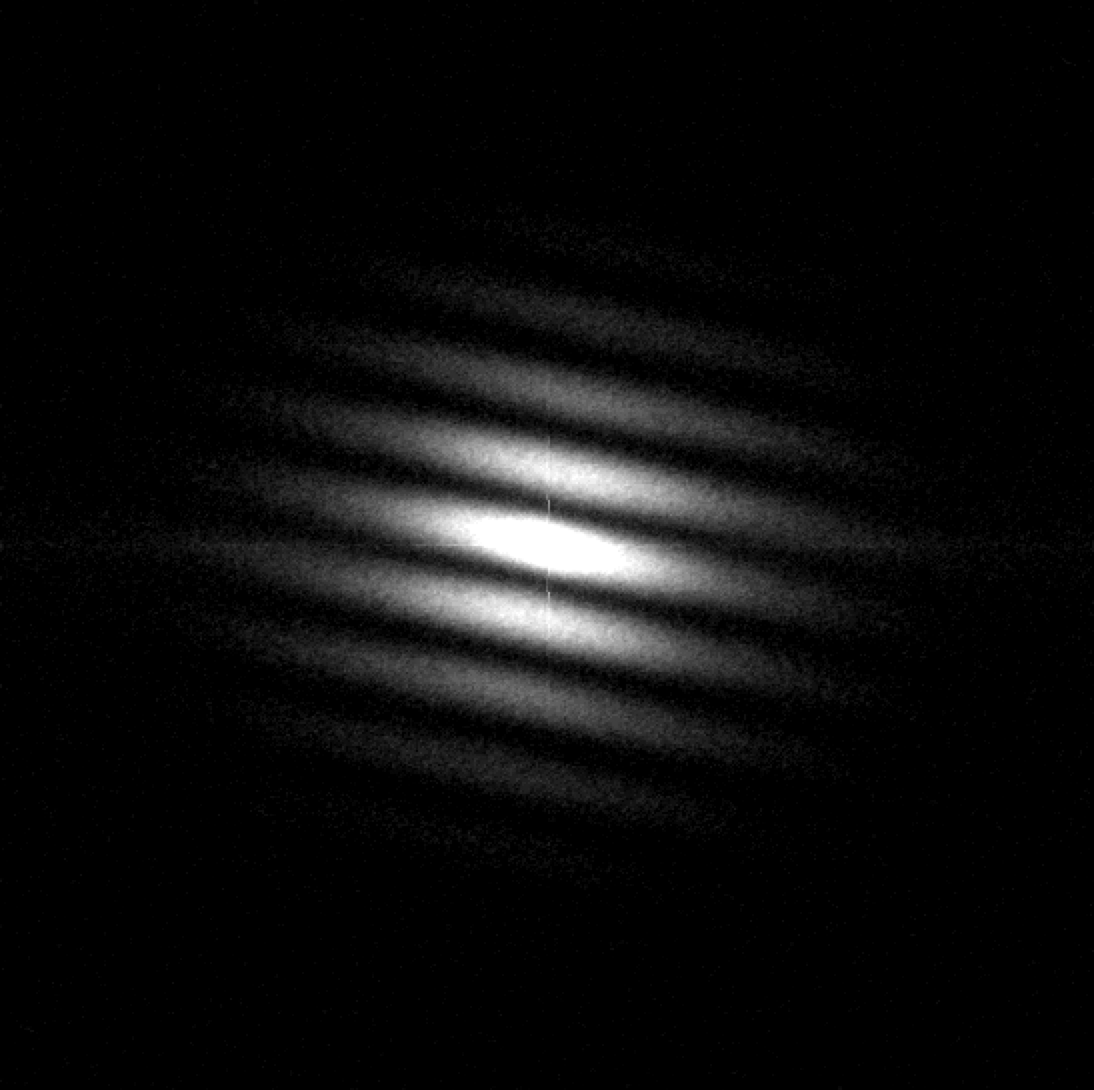# Reduction of speckle interferometric binary stars

## Workflow for reduction of speckle interferometric binary stars

For reduction of speckle images of binary and multiple stars we use algorithm described in paper Pluzhnik (2005).

Calculate statistics for object file

obj_filename <- file.choose() # Choose file with binary star
obj_stat <- speckle_stat(obj_filename)

Calculate statistics for dark file

dark_filename <- file.choose() # Choose file with dark frames
dark_stat <- speckle_stat(dark_filename)

Calculate statistics for flat file

flat_filename <- file.choose() # Choose file with flat field frames
flat_stat <- speckle_stat(flat_filename)

Calculate average dark frame

zero_matrix <- matrix(0, 512, 512)
mid_dark <- middle_frame(filename = dark_filename, subtrahend = zero_matrix)

Calculate average flat field frame with average dark subtraction

mid_flat <- middle_frame(flat_filename, subtrahend = mid_dark)

Calculate power spectrum with subtraction of neighboring frame

ps_diff <- speckle_ps_diff(obj_filename)

Calculate acf

acf <- speckle_acf(ps_diff)

Calculate power spectrum

ps <- speckle_ps(obj_filename, dark = mid_dark, flat = mid_flat)Fig. 1. Power spectrum of star ADS 15182 in 550/20 nm band.